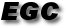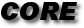Introduction
People
Papers
Talks
Wiki

##Introduction
Gallery/Videos
Features
CoreLib
Contact

Welcome to the homepage of the Exact Geometric Computation Group (EGC group).

We are in the Computer Science Department
of the Courant Institute for Mathematical Sciences
in NYU.

Our research goal is to understand the phenomenon of numerical non-robustness and to provide effective algorithmic solutions.
We use an approach called Exact Geometric Computation (EGC).
We validate our theory through the development of our software called Core Library.

Beyond EGC, we address the issues of guaranteed precision computation,
and by developing a theory of real approximation and its complexity.
We seek to develop the area of ``numerical computational geometry'', with emphasis on adaptive, topologically exact algorithms.
A major challenge here is the complexity analysis of adaptive algorithms.